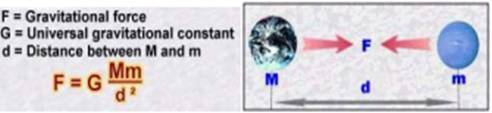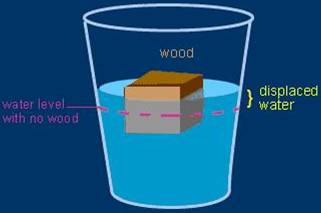# Gravitation class 9 Notes Science## myCBSEguide App

CBSE, NCERT, JEE Main, NEET-UG, NDA, Exam Papers, Question Bank, NCERT Solutions, Exemplars, Revision Notes, Free Videos, MCQ Tests & more.

CBSE class 9 Science Chapter 10 Gravitation notes in PDF are available for free download in myCBSEguide mobile app. The best app for CBSE students now provides Gravitation class 9 Notes latest chapter wise notes for quick preparation of CBSE exams and school based annual examinations. Class 9 Science notes on Chapter 10 Gravitation are also available for download in CBSE Guide website.

## CBSE Guide Gravitation class 9 Notes

CBSE guide notes are the comprehensive notes which covers the latest syllabus of CBSE and NCERT. It includes all the topics given in NCERT class 9 Science text book. Users can download CBSE guide quick revision notes from myCBSEguide mobile app and my CBSE guide website.

## 9 Science notes Chapter 10 Gravitation

Download CBSE class 9th revision notes for Chapter 10 Gravitation in PDF format for free. Download revision notes for Gravitation class 9 Notes and score high in exams. These are the Gravitation class 9 Notes prepared by team of expert teachers. The revision notes help you revise the whole chapter in minutes. Revising notes in exam days is on of the best tips recommended by teachers during exam days.

CBSE Class 9 Science
Revision Notes
CHAPTER – 10
GRAVITATION

Gravity is one of the most basic forces in the universe. It plays a fundamental role not only in the structure of our solar system but also in the way objects behave on Earth.

Gravitation

Gravitation is the force of attraction between two objects in the universe. Gravitation may be the attraction of objects by the earth.

This force is proportional to the product of masses of the objects and inversely proportional

to the square of the distance between them. It is independent of medium.

Gravitational force

Eg :- If a body is dropped from a certain height, it falls downwards due to earth’s gravity. If a body is thrown upwards, it reaches a certain height and then falls downwards due to the earth’s gravity.

• Gravitation may be the attraction between objects in outer space.

Eg :- Attraction between the earth and moon.

Attraction between the sun and planets.

GRAVITY

• A natural force that pulls all objects toward the center of the earth
• keeps the moon orbiting
• It holds stars together . . .
• And binds galaxies together for billions of years ….Prevents Planets from losing their atmospheres.

2.Universal law of gravitation:

• The universal law of gravitation states that, ‘Every object in the universe attracts every other object with a force which is directly proportional to product of the masses and inversely proportional to the square of the distance between them.’• The SI unit of G is  and its value is
• The strength of the gravitational attraction between two objects depends on two factors:
• How big the objects are (how much mass they have) and
• How far apart they are.

3. Free Fall

• With negligible air resistance, falling objects can be considered freely falling. objects of different shapes accelerate differently (stone vs feather)4 .To calculate the value of” g“(acceleration due to gravity)

• The acceleration due to gravity is denoted by g.
• The unit of g is same as the unit of acceleration
• From the second law of motion, force is the product of mass and acceleration. F = ma
• For free fall, force is the product of mass and acceleration due to gravity.

where M is the mass of the Earth and d is the distance between the object and the earth.

• For objects near or on the surface of the earth d is equal to the radius of the earth R

5. Mass and Weight:

• Mass is a fundamental, universal property. You have the same amount of mass no matter where you are in the Universe.
• Weight is not fundamental its value depends on circumstances in the Universe. Weight is a force. It is the resultant gravitational force exerted on a body with mass m by all the other bodies on the Universe.
Weight =
where is the mass of the Earth and R is the radius of the Earth.

6. Weight Of The Object On Moon

• The mass of the moon is less than the mass of the earth. So the moon exerts lesser force on the objects than the earth.
• The weight of an object on the moon is one sixth  of its weight on the earth.
• The weight of an object on the earth is the force with which the earth attracts the object and the weight of an object on the moon is the force with which the moon attracts the object.
 Celestial body Mass (kg) Radius (m) Earth 5 Moon

7. Thrust & Pressure

• Thrust is the force acting on an object perpendicular to the surface.
• Pressure is the force acting on unit area of a surface
• The SI unit of thrust is . It is called Pascal (Pa).

8. Pressure In Fluids

• Fluids exert pressure in all directions
• Pressure exerted on fluids is transmitted equally in all directions.

9. Buoyancy

• When an object is immersed in a fluid it experiences an upward force called buoyant force. This property is called buoyancy or upthrust.10. Why objects float or sink when placed on the surface of water?

• Take some water in a beaker. Take a piece of cork and an iron nail of the same mass. Place them on the water. The cork floats and the nail sinks.
• If the density of an object is less than the density of a liquid, it will float on the liquid and if the density of an object is more than the density of a liquid, it will sink in the liquid.

11. Archeimedes Principle

• Archimedes’ principle states that, When a body is partially or fully immersed in a fluid it experiences an upward force that is equal to the weight of the fluid displaced by it.’

12 .Relative density

• The relative density of a substance is the ratio of the density of a substance to the density of water. It is a ratio of similar quantities and has no unit.

## Gravitation class 9 Notes

• CBSE Revision notes for Class 9 Science PDF
• CBSE Revision notes Class 9 Science – CBSE
• CBSE Revisions notes and Key Points Class 9 Science
• Summary of the NCERT books all chapters in Science class 9
• Short notes for CBSE class 9th Science
• Key notes and chapter summary of Science class 9
• Quick revision notes for CBSE exams

## CBSE Class-9 Revision Notes and Key Points

Gravitation class 9 Notes. CBSE quick revision note for Class-9 Science, Chemistry, Maths, Biology and other subject are very helpful to revise the whole syllabus during exam days. The revision notes covers all important formulas and concepts given in the chapter. Even if you wish to have an overview of a chapter, quick revision notes are here to do if for you. These notes will certainly save your time during stressful exam days.

To download Gravitation class 9 Notes, sample paper for class 9 Mathematics, Social Science, Science, English Communicative; do check myCBSEguide app or website. myCBSEguide provides sample papers with solution, test papers for chapter-wise practice, NCERT solutions, NCERT Exemplar solutions, quick revision notes for ready reference, CBSE guess papers and CBSE important question papers. Sample Paper all are made available through the best app for CBSE students and myCBSEguide website.